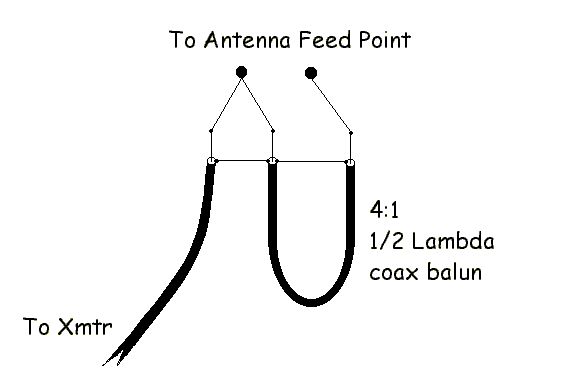Here we calculate the half wave length of the matching section of the 4:1 coax balun. Again the equations are taken from the ARRL Antenna Book.

### Output Values

Half Lambda Coax Matching Section Length (Ft.) =
Half Lambda Coax Matching Section Length (In.) =

### Input Values

Change the value, move the cursor to empty space and click
to re-calculate.
Frequency (MHz) =
Velocity Factor (decimal percent) =